STRATA Statement

STRATA variable <(list)> <…variable <(list)>> </ options> ;

The STRATA statement identifies the variables that determine the strata levels. Strata are formed according to the nonmissing values of these variables. The MISSING option can be used to allow missing values as a valid stratum level. Other options enable you to specify various k-sample tests, stratified tests, or trend tests and to make multiple-comparison adjustments for paired differences.

In the preceding syntax, variable is a variable whose values determine the stratum levels, and list is a list of endpoints for a numeric variable. The values for variable can be formatted or unformatted. If variable is a character variable, or if variable is numeric and no list appears, then the strata are defined by the unique values of the STRATA variable. More than one variable can be specified in the STRATA statement, and each numeric variable can be followed by a list. Each interval contains its lower endpoint but not its upper endpoint. The corresponding strata are formed by the combination of levels. If a variable is numeric and is followed by a list, then the levels for that variable correspond to the intervals defined by the list. The initial interval is assumed to start at, and the final interval is assumed to end at.

The specification of a STRATA variable can have any of the following forms:A list separated by blanks Age(5 10 20 30)A list separated by commas Age(5,10,20,30)x to y Age(5 to 10)x to y by z Age(5 to 30 by 10)A combination of the above Age(5,10 to 50 by 10)

For example, the specification

strata Age(5,20 to 50 by 10) Sex;

indicates the following levels for the Age variable: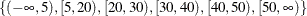This statement also specifies that the Age strata be further subdivided by values of the variable Sex. In this example, there are six age groups by two sex groups, forming a total of 12 strata.

The specification of several STRATA variables, such as

strata A B C;

is equivalent to the A*B*C syntax of the TABLES statement in the FREQ procedure. The number of strata levels usually grows very rapidly with the number of STRATA variables, so you must be cautious when specifying the list of STRATA variables.

When comparing more than two survival curves, a k-sample test tells you whether the curves are significantly different from each other, but it does not identify which pairs of curves are different. A multiple-comparison adjustment of the p-values for the paired comparisons retains the same overall false positives as the k-sample test. Two types of paired comparisons can be made: comparisons between all pairs of curves and comparisons between a control curve and all other curves. You use the DIFF= option to specify the comparison type, and you use the ADJUST= option to select a method of multiple-comparison adjustments.

Table 52.3 summarizes the options available in the STRATA statement.

Table 52.3: Options Available in the STRATA Statement

Option

Description

Homogeneity Tests

Specifies the group variable for stratified tests

Suppresses printing the test statistic and covariance matrix

Suppresses any tests

Specifies tests corresponding to various weight functions

Requests a trend test

Multiple Comparisons

Specifies the type of differences to consider

Missing Strata Value

Allows missing values as valid stratum values

Display Option

Uses the names of the STRATA variables in the display

You can specify the following options in the STRATA statement after a slash (/).

specifies the multiple-comparison method for adjusting the p-values of the paired tests. See the section Multiple-Comparison Adjustments for mathematical details; also see Westfall et al. (1999). The adjustment methods include the following:

BONFERRONI
BON

applies the Bonferroni correction to the raw p-values.

DUNNETT

performs Dunnett’s two-tailed comparisons of the control group with all other groups. PROC LIFETEST uses the factor-analytic covariance approximation described in Hsu (1992) and identifies the adjustment in the results as Dunnett-Hsu. Note that ADJUST=DUNNETT is incompatible with DIFF=ALL.

SCHEFFE

SIDAK

applies the Šidák correction to the raw p-values.

SMM
GTE

performs the paired comparisons based on the studentized maximum modulus test.

TUKEY

performs the paired comparisons based on Tukey’s studentized range test. PROC LIFETEST uses the approximation described in Kramer (1956) and identifies the adjustment as "Tukey-Kramer" in the results. Note that ADJUST=TUKEY is incompatible with DIFF=CONTROL.

SIMULATE <(simulate-options)>

computes the adjusted p-values from the simulated distribution of the maximum or maximum absolute value of a multivariate normal random vector. The simulation estimates q, the true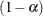quantile, where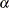is the value of the ALPHA= simulate-option.

The number of samples for the SIMULATE adjustment is set so that the tail area for the simulated q is within a certain accuracy radiusofwith an accuracy confidence of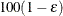%. In equation form,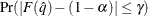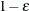where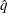is the simulated q and F is the true distribution function of the maximum; see Edwards and Berry (1987) for details. By default,= 0.005 and= 0.01 so that the tail area ofis within 0.005 of 0.95 with 99% confidence.

The simulate-options include the following:

ACC=value

specifies the target accuracy radiusof a% confidence interval for the true probability content of the estimatedquantile. The default value is ACC=0.005.

ALPHA=value

specifies the valuefor estimating thequantile. The default value is the ALPHA= value in the PROC LIFETEST statement, or 0.05 if that option is not specified.

EPS=value

specifies the valuefor a% confidence interval for the true probability content of the estimatedquantile. The default value for the accuracy confidence is 99%, corresponding to EPS=0.01.

NSAMP=n

specifies the sample size for the simulation. By default, n is set based on the values of the target accuracy radiusand accuracy confidence% for an interval for the true probability content of the estimatedquantile. With the default values for,, and(0.005, 0.01, and 0.05, respectively), NSAMP=12604 by default.

REPORT

specifies that a report on the simulation should be displayed, including a listing of the parameters, such as,, and, in addition to an analysis of various methods for estimating or approximating the quantile.

SEED=number

specifies an integer used to start the pseudorandom number generator for the simulation. If you do not specify a seed, or if you specify a value less than or equal to zero, the seed is generated by default from reading the time of day from the computer’s clock.

DIFF=ALL | CONTROL<(’string’ <…, ’string’>)>

specifies which pairs of survival curves are considered for the multiple comparisons.

DIFF=ALL

requests all paired comparisons

DIFF=CONTROL <(’string’ <…’string’>)>

requests comparisons of the control curve with all other curves. To specify the control curve, you specify the quotes strings of formatted values that represent the curve in parentheses. For example, if Cell=’large’ identifies the control group, you specify

DIFF=CONTROL('large')

If more than one variable is used to identify the curves (for example, if Cell=’large’ and Sex=’F’ represent the control), you specify

DIFF=CONTROL('large' 'F')

The order of the quoted strings should correspond to the order of the stratum variables. If no specific curve is specified as the control, the first stratum or group value is used.

By default, DIFF=ALL unless you specify ADJUST= DUNNETT, in which case DIFF=CONTROL.

GROUP=variable

specifies the variable whose formatted values identify the various samples whose underlying survival curves are to be compared. The tests are stratified on the levels of the STRATA variables. For example, in a multicenter trial in which two forms of therapy are to be compared, you specify the variable that identifies therapies as the GROUP= variable and the variable that identifies centers as the STRATA variable, in order to perform a stratified test to compare the therapies while controlling the effect of the centers.

MISSING

allows missing values to be a stratum level or a valid value of the GROUP= variable.

NODETAIL

suppresses the display of the rank statistics and the corresponding covariance matrices for various strata. If you specified the TREND option, the display of the scores for computing the trend tests is suppressed.

NOLABEL

specifies that the names instead of the labels of the STRATA variables be used in the display of the survival estimate table and in the legend of the survival plot.

NOTEST

suppresses the k-sample tests, stratified tests, and trend tests.

ORDER=FORMATTED | INTERNAL

specifies the sorting order of the values of the STRATA variables. The strata are presented in the specified order in the analysis results. You can use this option, for example, to display the curve labels in your preferred order in the survival plot legend (see Example 52.2 for an illustration). The default is ORDER=FORMATTED, which sorts the strata according to their external formatted values, except for numeric variable with no explicit format, which are sorted by the unformatted (internal) values. ORDER=INTERNAL sorts the strata by their internal values. The ORDER= option has no effect on a stratum variable with cutpoints specified.

TREND

computes the trend tests for testing the null hypothesis that the k population hazards rate are the same versus an ordered alternatives. If there is only one STRATA variable and the variable is numeric, the unformatted values of the variable are used as the scores; otherwise, the scores are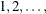in the given order of the strata.

TEST=test-request
TEST=(test-request <…test-request> )

controls the tests produced. Each test corresponds to a different weight function (see the section Nonparametric Tests for the weight functions). The test-requests include the following:

ALL

specifies all the nonparametric tests with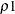=1 and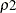=0 for the Fleming and Harrington test—FLEMING(1,0).

FLEMING(,)

specifies the family of tests in Harrington and Fleming (1982), whereandare nonnegative numbers. FLEMING(,) reduces to the Fleming-Harrington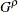family (Fleming and Harrington, 1981) when=0, which you can specify as FLEMING(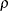) with one argument. When=0, the test becomes the log-rank test. When=1, the test should be very close to the Peto-Peto test.

LOGRANK

specifies the log-rank test.

NONE

suppresses all comparison tests. Specifying TEST=NONE is equivalent to specify NOTEST.

LR

specifies the likelihood ratio test based on the exponential model.

MODPETO

specifies the modified Peto-Peto test.

PETO

specifies the Peto-Peto test. The test is also referred to as the Peto-Peto-Prentice test.

WILCOXON

specifies the Wilcoxon test. The test is also referred to as the Gehan test or the Breslow test.

TARONE

specifies the Tarone-Ware test.

By default, TEST=(LOGRANK WILCOXON LR) for the k-sample tests, and TEST=(LOGRANK WILCOXON) for stratified and trend tests.